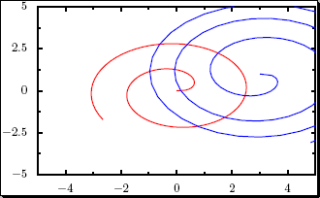## Titus' PyX Tutorial for Gnuplot Users

By Titus Winters

Translations:
Belarusian
Estonian
Russian

Note: This tutorial assumes you have basic familiarity with both Gnuplot and the Python programming language. Without some understanding of those, you're going to be quite lost.

At some point, it is bound to happen. Gnuplot is wonderful, but there comes a time where it just doesn't quite have the power that you need it to have. Perhaps you want to radically alter the way the axes are drawn. Perhaps you just want to do something simple like change the color of a plot line, but not the pattern. Maybe you really need some hefty math symbols displayed on the graph. At some point you'll hit the wall beyond which Gnuplot quickly stops being the right answer. What works better in these situations?

There are many answers. HippoDraw is powerful and free, although relatively unknown. Some people like XGraph. If you, like me, are a Python programmer, then you might be willing to go for a less user-friendly but more controllable solution. If so, then PyX (Pronounced "Piks") is one possibility.

As of this writing (January 2006), PyX is still pre-release software. Advertising a version of 0.8.1, there are definitely some rough edges on this. Usage of PyX may very well require some significant poking at the Python objects that make up the PyX framework. However, I can attest that this is time well spent: it took about 3 or 4 hours to go from first installing PyX to generating graphs that were (in my opinion) greatly superior to what I could have gotten out of Gnuplot, my normal go-to for graphing.

Basic Usage
Multiple Plots
Plot Styles
Error Bars
Axis Labels and Scales
Graph Titles
Plotting Functions
Closing Notes

### Basic Usage

Probably the most common thing to do in Gnuplot is to plot a simple data file. (Sample data files are available
here and here).
gnuplot> plot "data.dat"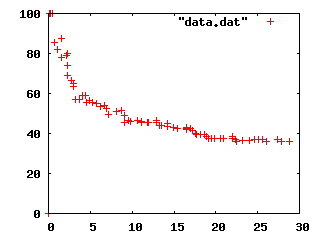How do we do the equivalent in PyX? This depends on how equivalent you want to be: the defaults in these two environments are different. So we'll first show the simplest graph for the same available in PyX, and then follow with making the graph look like the Gnuplot equivalent.
from pyx import *

g = graph.graphxy(width=8)
g.plot(graph.data.file("data.dat", x=1, y=2))
g.writePDFfile("test")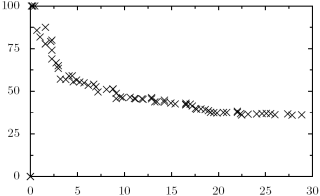Note: PyX does not have any display capabilities, so you'll need to write to PDF or EPS and then use you favorite viewer for those file formats.

There are three major differences between these two plots:
1. Color - Gnuplot defaults to printing the first plot in red, the second in green, etc. (BTW: This is unfortunate for the ~3% of the population that is Red-Green colorblind.) PyX defaults to black and white.
2. Key - Gnuplot defaults to rendering a key for each line in a plot, PyX does not.
3. Image size - Gnuplot defaults to old computer-monitor resolutions like 640x480 with a ratio of 1.33:1. PyX defaults to canvases with the Golden Ratio of ~1.618:1. (This is likely to be less important to most, but I include it for completeness.)
Now we shall address these, one at a time.

#### Key

Adding a key is very simple. Simply ask the graphxy object to include one:
from pyx import *

g = graph.graphxy(width=8, key=graph.key.key())
g.plot(graph.data.file("data.dat", x=1,y=2))
g.writePDFfile("test")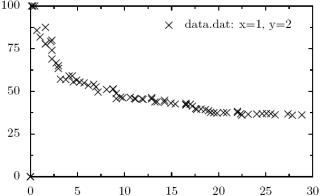In truth, it is unlikely that you want the default title for the data line that is provided in such a plot. Setting the title in gnuplot would be:
gnuplot> plot "data.dat" title "My Data"

Similarly in PyX:
from pyx import *

g = graph.graphxy(width=8, key=graph.key.key())
g.plot(graph.data.file("data.dat", x=1, y=2, title="My Data"))
g.writePDFfile("test")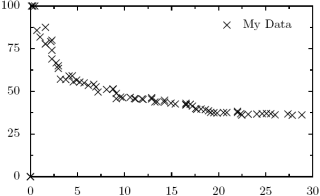Note: Text in PyX is being typeset by LaTeX. This has advantages and disadvantages. If you wish for your title to include math symbols, Greek characters, etc, then simply enter the correct LaTeX formula. On the other hand, if you want to use the "%" sign, be careful: that is a comment in LaTeX and thus you will cause some significant confusion to PyX. Another thing to be watchful of is that Python may interpret LaTeX symbols incorrectly: "\tau" in Python is going to be interpreted as "\t" (the tab character) followed by "au". A good solution for this is the "raw string" feature in Python: preface your strings with 'r': r"\tau", then no escape character expansion will be performed.

#### Ratio

If you really want the ratio of your graph dictated by monitor resolutions from the 90s, rather than Greek architecture, you need to alter the ratio of the graph object. (I'd suggest leaving the default.)
from pyx import *

g = graph.graphxy(width=8, ratio=4./3, key=graph.key.key())
g.plot(graph.data.file("data.dat", x=1, y=2, title="My Data"))
g.writePDFfile("test")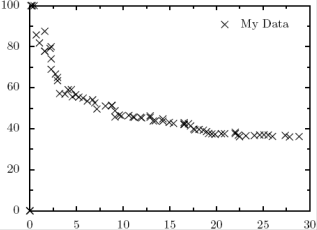#### Color

Altering the color is in fact altering the plot style. The setup that most closely mimics Gnuplot's defaults is something like this:
from pyx import *

g = graph.graphxy(width=8, ratio=4./3, key=graph.key.key())
g.plot(graph.data.file("data.dat", x=1, y=2, title="My Data"),
g.writePDFfile("test")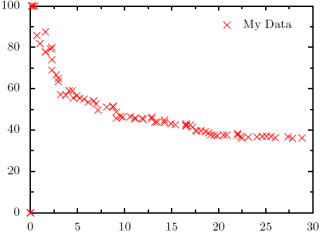Here we are providing a list of styles. Styles are special objects for PyX, found in the graph module. (Use of dir and help from the Python command line can be very instructive here.) In this case the only thing we are providing is an instruction to use symbols. The constructor for symbol can take a named argument, symbolattrs, which again takes a list of attributes that can be used to change the display of the symbol. In this case we are providing a gradient object. The gradient object's job is to return a color to draw the next line in. Most gradients are a simple two-color gradient (do a dir() on color.gradient to see the predefined options), but Rainbow is slightly more tricky than that, and produces results in more varied colors. It won't mimic Gnuplot's color choices entirely, but that really isn't bad. (I mean really: the 6th color in Gnuplot is yellow?)

### Multiple Plots

OK, so we can mimic a simple, single-plot Gnuplot graph. Moving on to multiple plots.
gnuplot> plot "data.dat" title "Data 1", "data2.dat" title "Data 2"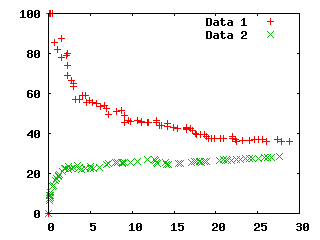Multiple plots in PyX is equivalently simple:
from pyx import *

# Initialize graph object
g = graph.graphxy(width=8, ratio=4./3, key=graph.key.key())

# These are the data lines we want to plot.
data = [graph.data.file("data.dat", x=1, y=2, title="Data 1"),
graph.data.file("data2.dat", x=1, y=2, title ="Data 2")]

# Plot it
g.plot(data,

# Write the output
g.writePDFfile("test")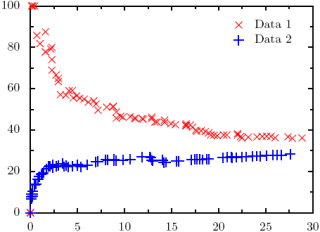### Plot styles

How about changing plot styles from points to lines or linespoints?
gnuplot> plot "data.dat" title "Data 1" with lines, "data2.dat" title "Data 2" with linespoints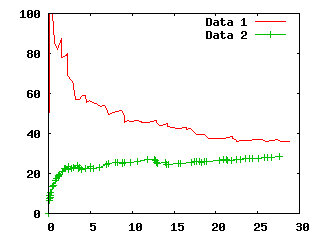This gets a little more complicated, but not too bad. We return to plotting one line at a time. This is not the most simple invocation for doing this (I'm adding in a few extra options), but I am taking this opportunity to show off a few extra features that you ought to be able to generalize from.

from pyx import *

# Initialize graph object
g = graph.graphxy(width=8, ratio=4./3, key=graph.key.key())

# Plot the first line
g.plot(graph.data.file("data.dat", x=1, y=2, title="Data 1"),
styles=[graph.style.line([color.rgb.red,
style.linestyle.solid,
style.linewidth.thick])])

# Plot the second line
g.plot(graph.data.file("data2.dat", x=1, y=2, title ="Data 2"),
styles=[graph.style.line([color.rgb.green,
style.linestyle.solid,
style.linewidth.thick]),
graph.style.symbol(symbolattrs=[color.rgb.green])])

# Write the output
g.writePDFfile("test")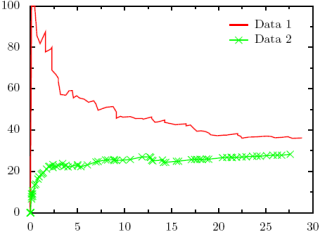### Error Bars

Adding error bars in PyX is quite easy, and gives you much greater control over how the points are drawn (with or without symbols, different color error bars vs. symbols, size of symbol, etc).

This section uses
this as a data file.
plot "error.dat" using 1:2:3 with yerrorbars title "Samples"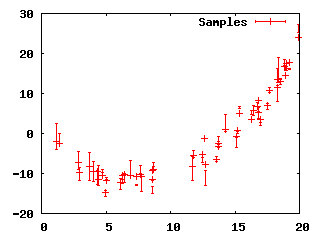from pyx import *

g = graph.graphxy(width=8)

g.plot(graph.data.file("error.dat", x=1, y=2, dy=3),
styles=[graph.style.errorbar(errorbarattrs=[color.rgb.red]),
graph.style.symbol(graph.style.symbol.circle, size=0.05,
symbolattrs=[color.rgb.red])])

g.writePDFfile("test.pdf")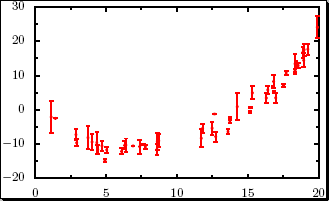### Axis Labels and Scales

Again, common and easy features in Gnuplot that turn out to be almost as easy in PyX. Lets add labels to our graphs so we know what the axes represent.
gnuplot> set ylabel "# Roaches"
gnuplot> set xlabel "Time (days)"
gnuplot> plot "data.dat" title "Data 1" with lines, "data2.dat" title "Data 2" with linespoints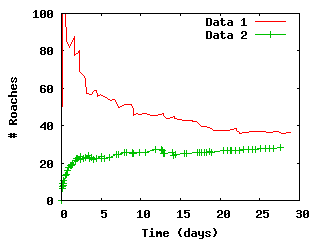And the equivalent in PyX. The only thing to watch out for here is the calls down to LaTeX.

from pyx import *

# Initialize graph object
g = graph.graphxy(width=8, ratio=4./3, key=graph.key.key(),
x=graph.axis.linear(title="Time (days)"),
y=graph.axis.linear(title="$\#$ Roaches"))

# Plot the first line
g.plot(graph.data.file("data.dat", x=1, y=2, title="Data 1"),
styles=[graph.style.line([color.rgb.red,
style.linestyle.solid,
style.linewidth.thick])])

# Plot the second line
g.plot(graph.data.file("data2.dat", x=1, y=2, title ="Data 2"),
styles=[graph.style.line([color.rgb.green,
style.linestyle.solid,
style.linewidth.thick]),
graph.style.symbol(symbolattrs=[color.rgb.green])])

# Write the output
g.writePDFfile("test")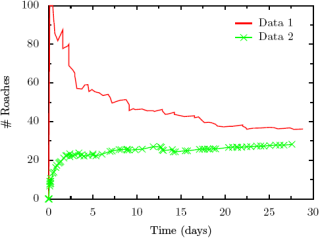How about if we want to bump the ranges for the plots a little?
gnuplot> set ylabel "# Roaches"
gnuplot> set xlabel "Time (days)"
gnuplot> set xrange [0 to 35]
gnuplot> set yrange [0 to 105]
gnuplot> plot "data.dat" title "Data 1" with lines, "data2.dat" title "Data 2" with linespoints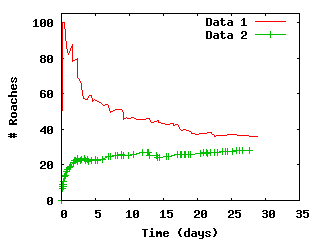Again, in PyX, very easy.

from pyx import *

# Initialize graph object
g = graph.graphxy(width=8, ratio=4./3, key=graph.key.key(),
x=graph.axis.linear(min=0, max=35, title="Time (days)"),
y=graph.axis.linear(min=0, max=105, title="$\#$ Roaches"))

# Plot the first line
g.plot(graph.data.file("data.dat", x=1, y=2, title="Data 1"),
styles=[graph.style.line([color.rgb.red,
style.linestyle.solid,
style.linewidth.thick])])

# Plot the second line
g.plot(graph.data.file("data2.dat", x=1, y=2, title ="Data 2"),
styles=[graph.style.line([color.rgb.green,
style.linestyle.solid,
style.linewidth.thick]),
graph.style.symbol(symbolattrs=[color.rgb.green])])

# Write the output
g.writePDFfile("test")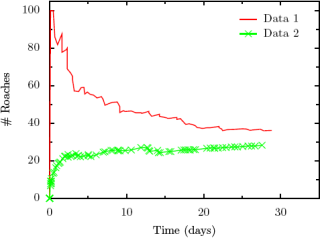### Graph Titles

Definitely one of the least-elegant Gnuplot-equivalents for PyX, adding a title to a graph is actually making use of PyX's capability to add text anywhere on a graph. Don't like the default key? Make a new one. Want to add labels right onto a graph? Do it. Don't want vertical text for your y-label? Do it by hand. The ability to typeset text right onto your graph can be very handy, but that comes with a price: it is a little clunky from time to time.
gnuplot> set ylabel "# Roaches"
gnuplot> set xlabel "Time (days)"
gnuplot> set xrange [0 to 35]
gnuplot> set yrange [0 to 105]
gnuplot> set title "Effectiveness of Brand X Roach Bait"
gnuplot> plot "data.dat" title "Data 1" with lines, "data2.dat" title "Data 2" with linespoints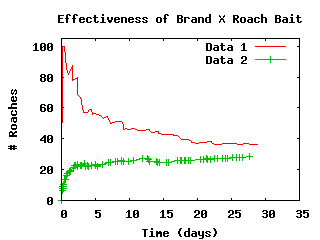from pyx import *

# Initialize graph object
g = graph.graphxy(width=8, ratio=4./3, key=graph.key.key(),
x=graph.axis.linear(min=0, max=35, title="Time (days)"),
y=graph.axis.linear(min=0, max=105, title="$\#$ Roaches"))

# Plot the first line
g.plot(graph.data.file("data.dat", x=1, y=2, title="Data 1"),
styles=[graph.style.line([color.rgb.red,
style.linestyle.solid,
style.linewidth.thick])])

# Plot the second line
g.plot(graph.data.file("data2.dat", x=1, y=2, title ="Data 2"),
styles=[graph.style.line([color.rgb.green,
style.linestyle.solid,
style.linewidth.thick]),
graph.style.symbol(symbolattrs=[color.rgb.green])])

# Now plot the text, horizontally centered
g.text(g.width/2, g.height + 0.2, "Effectiveness of Brand X Roach Bait",
[text.halign.center, text.valign.bottom, text.size.Large])

# Write the output
g.writePDFfile("test")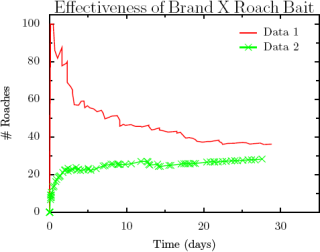### Plotting Functions

One example that a reader contributed was graphing points defined by a file but modified functionally. For example, in Gnuplot:
plot "data.dat" title "Data 1" with lines title "Data 1"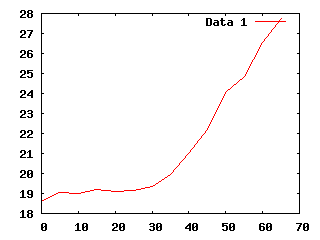And it is roughly the same in PyX (thanks to Kipton Barros for the tip):
from pyx import *

# Initialize graph object
g = graph.graphxy(width=8, ratio=4./3, key=graph.key.key())
g.plot(graph.data.file("data.dat", x=1, y="\$2/2", title="Data 1"),
styles=[graph.style.line([color.rgb.red,
style.linestyle.solid,
style.linewidth.thick])])
g.writePDFfile("test")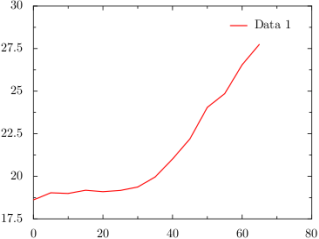Another common use for Gnuplot is to plot a function, be it purely mathematical or a best-fit for your data. (It is worth noting that PyX has no substitute for Gnuplot's "fit" capabilities if you are working with best-fit functions.)
set xrange [0:10]
set yrange [0:10]

f(x) = .2 * x**2 - x + 1
plot f(x)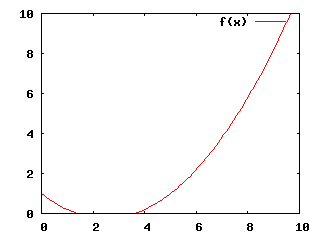The equivalent in PyX is easy if you've been following along, although certainly more typing.
from pyx import *

# Initialize graph object
g = graph.graphxy(width=8,
key=graph.key.key(),
x=graph.axis.linear(min=0, max=10),
y=graph.axis.linear(min=0, max=10))

# Plot the function
g.plot(graph.data.function("y(x) = .2 * x**2 - x + 1"),
styles=[graph.style.line([color.rgb.red,
style.linestyle.solid,
style.linewidth.thick])])

# Write pdf
g.writePDFfile("test.pdf")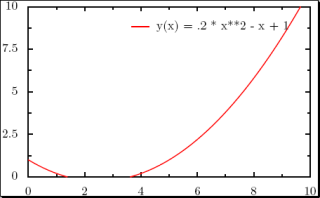set parametric

set xrange[-5:5]
set yrange[-5:5]
set trange[0:10]
unset key

x(t) = cos(t) * t**.5
y(t) = sin(t) * t**.5

plot x(t),y(t)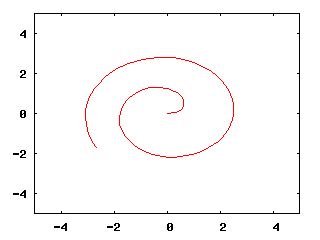In PyX, we start to see some of the power of Python showing through.
import math

def x(k):
return math.cos(k) * k**.5

def y(k):
return math.sin(k) * k**.5

# Initialize graph object
g = graph.graphxy(width=8,
x=graph.axis.linear(min=-5, max=5),
y=graph.axis.linear(min=-5, max=5))

# Plot the function
kMin = 0
kMax = 10

# The "context" parameter is a Python context, allowing us
# to use functions locally defined in this function. OR
# we can make the string "x, y = x(k), y(k)" into a more complex
# Python expression (it is being passed to eval() under the hood.)
g.plot(graph.data.paramfunction("k", kMin, kMax,
"x, y = x(k), y(k)",
context=locals()),
styles=[graph.style.line([color.rgb.red,
style.linestyle.solid,
style.linewidth.thick])])

# Write pdf
g.writePDFfile("test.pdf")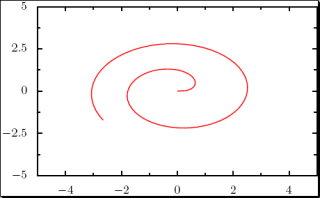Or how about something that Gnuplot can't do: multiple parametric curves with differing ranges? (Gnuplot only allows one range for the parametric value t at a time, so all functions being plotted must be written with the same range in mind. PyX has no such limitation.)
import math

def x(k):
return math.cos(k) * k**.5

def y(k):
return math.sin(k) * k**.5

# Initialize graph object
g = graph.graphxy(width=8,
x=graph.axis.linear(min=-5, max=5),
y=graph.axis.linear(min=-5, max=5))

# Plot the function
kMin = 0
kMax = 10
g.plot([graph.data.paramfunction("k", kMin, kMax,
"x, y = x(k), y(k)",
context=locals()),
graph.data.paramfunction("k", 0, 20,
"x, y = 3+x(k), 1-y(k)",
context=locals())],